# SBI PO Reasoning Questions 2019 (Day-44) High Level-New Pattern

Dear Aspirants, Our IBPS Guide team is providing new series of Reasoning Questions for SBI PO 2019 so the aspirants can practice it on a daily basis. These questions are framed by our skilled experts after understanding your needs thoroughly. Aspirants can practice these new series questions daily to familiarize with the exact exam pattern and make your preparation effective.

[WpProQuiz 6649]

(Directions 1–5): Study the following information carefully and answer the questions given below it.

‘A & B’ means ‘A is mother of B’.

‘A % B’ means ‘A is father of B’.

‘A # B’ means ‘A is sister of B’.

‘A @ B’ means ‘A is brother of B’.

‘A ^ B’ means ‘A is son of B’.

‘A * B’ means ‘A is daughter of B’.

1) In the given expression below which of the following statement is not true?

[email protected]&B#D; [email protected]&U; D%R#T^M; L*[email protected]*F;

a) B is aunty of T.

b) D is son-in-law of G.

c) R is daughter-in-law of F.

d) M is niece of K.

e) All the given statements are true.

2) How H is related to U in the given expression given below?

[email protected]&W*G%T&U^L

a) Father-in-law

b) Maternal Grandmother

c) Sister-in-law

d) Maternal Aunty

e) None of these

3) In which of the following expression M is maternal Uncle of H?

a) D^R#W%[email protected]%[email protected]*U

b) R*D%M#[email protected]&[email protected]^U

c) [email protected]&D#[email protected]%K#H^U

d) R#[email protected]&[email protected]&L#H^U

e) None of these

4) Which of the following option correctly fill the blanks so that D is father-in-law of H?

F#D__U__T*R__B%K__H

a) &, @, %, *

b) #, %, @, *

c) %, @, &, ^

d) &, *, %, ^

e) None of these

5) How U is related to V in the given expression given below?

R%L^U#[email protected]&H#V

a) Mother-in-law

b) Maternal Aunty

c) Father-in-law

d) Grand Father

e) None of these

(Directions 6–10): Study the following information carefully and answer the questions given below it.

P^Q means–P is 2m North of Q.

P%Q means–P is 2m East of Q.

[email protected] means–P is 2m South of Q.

P#Q means–P is 2m West of Q.

Also,

P ^% Q means–P is North-East of Q and so on based on the above directions.

6) In the given expression “P^Q#R; U^T%S; [email protected]” what is the position of U with respect to P?

a) East

b) North-East

c) South-East

d) North-West

e) Can’t be determined.

7) In the given expression “A#B^C; E#[email protected]; [email protected]%C; H%G” what is the shortest distance between H and C?

a) 6m

b) 2√2m

c) 8m

d) 2√3m

e) None of these

8) In the given expression “C%B^A; F#G^H; D^E; [email protected]#D; [email protected]%C” what is the position of A with respect to H?

a) South-east

b) East

c) North-east

d) North-west

e) None of these

9) What is the area of region enclosed by joining a line from A to F in the given expression “C%B^A; F#G^H; D^E; [email protected]#D; [email protected]%C”?

a) 16m2

b) 12√2m2

c) 10m2

d) 12m2

e) Can’t be determined.

10) What is the position of B with respect to E in the given expression “A^B#C; E#F^G; [email protected]%D^C”?

a) East

b) South-west

c) South-east

d) West

e) Can’t be determined.

Direction (1-5) :

We have:

[email protected]&B#D; [email protected]&U; D%R#T^M; L*[email protected]*F;

From above given expression we have: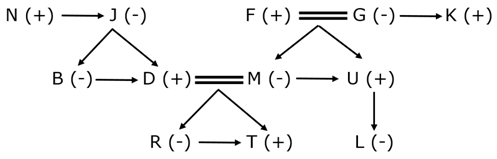We have:

[email protected]&W*G%T&U^L

From above given expression we have: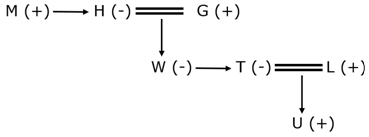Clearly, H is maternal grandmother of U.

Hence, option B is correct choice.

We have:

From option D we have: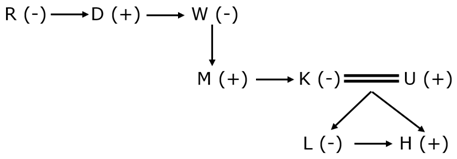Hence, option D is correct choice.

From option C we have: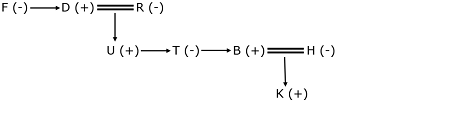Clearly, D is father-in-law of H.

Hence, option C is correct choice.

We have:

R%L^U#[email protected]&H#V

From above given expression we have: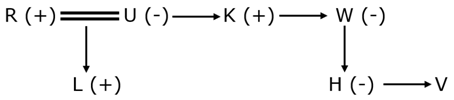Clearly, U is maternal aunty of V.

Hence, option B is correct choice.

Direction (6-10) :

We have:

P^Q#R; U^T%S; [email protected]

After combining above statements we have: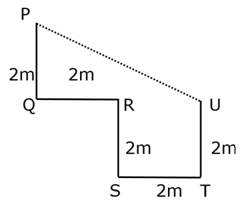Clearly, U is south-east of P.

Hence, option C is correct choice.

We have:

A#B^C; E#[email protected]; [email protected]%C; H%G

After combining above statements we have: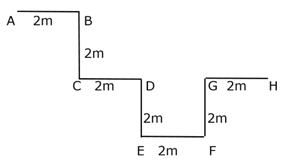Clearly, shortest distance between C to H is 6m.

Hence, option A is correct choice.

We have:

C%B^A; F#G^H; D^E; [email protected]#D; [email protected]%C

After combining above statements we have: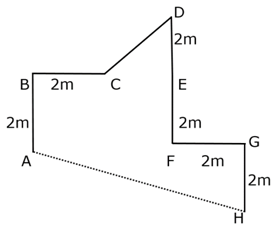Clearly, A is north-west of H.

Hence, option D is correct choice.

We have:

B^A#C; F#G^H; D^E; [email protected]#D; [email protected]

After combining above statements we have: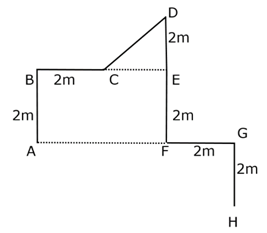Since, B is west of E.

Thus, CD = 2√2 and CE = 2m

Area of ABCEF = area (ABEF) + area (CDE)

Thus, area (ABEF) = (2 x 4) = 8m

Area (CDE) = ½ x 2 x 2 = 2m

Thus, required area = (8 + 2) = 10m2

Hence, option C is correct choice.

We have:

A^B#C; E#F^G; [email protected]%D^C

After combining above statements we have: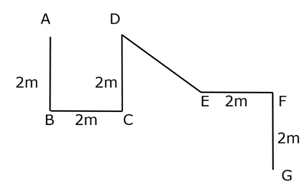Since, exact length of DE is not known and there is no direct relation between CE is known.

Thus, we can’t say exact direction of E with respect to B.

Hence, option E is correct choice.